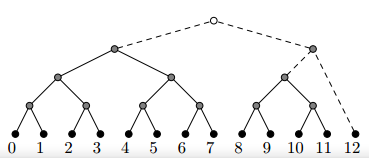# 2010 NEERC Western subregional

## 2010 NEERC Western subregional

solution

### Problem C. Canonical Binary Treesolution
$n$的二进制有多少个$1$就有多少棵树，而且每个$1$对应的位置就是对应的树的叶子节点数，按照这个很容易就能解决问题。

solution

### Problem E. Express Lines

solution
$dp$，把环拆成链，$f[i][0$~$1][0$~$1]$表示到第$i$个点，开头的点有没有选，第$i$个点有没有选的方案。答案等于$f[n]+f[n]+f[n]$

solution

### Problem G. Game

solution

$P(A|B)=\frac{P(AB)}{P(B)}=\frac{2(k)(m)-k^2}{2(k)(2m)-k^2}=\frac{2m-k}{4m-k}$

solution

### Problem M. Munich

solution
$dp$$f[i][j]$表示$i$个成人，$j$个儿童已经买了票的最少花费，然后按题意转移就好。

posted @ 2018-09-13 00:01  GerynOhenz  阅读(202)  评论(0编辑  收藏